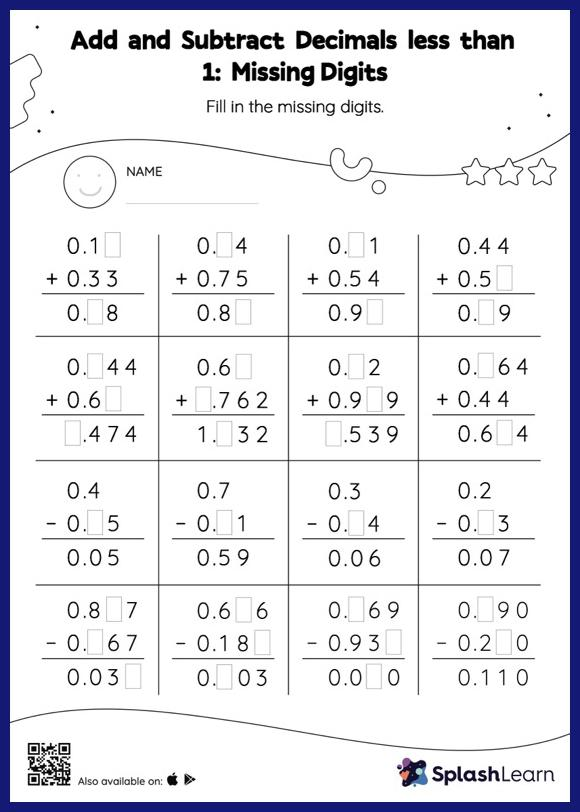# Add and Subtract Decimals less than 1: Missing Digits Worksheet

Home > Add and Subtract Decimals less than 1: Missing DigitsAdd and subtract decimals less than 1 worksheet helps students develop proficiency with addition and subtraction. In this worksheet, students add and subtract decimals by aligning the decimal points and using zero as a placeholder. They then use the relationship between addition and subtraction to find the missing number in the add and subtract decimals less than 1 worksheet.